Next: Lubrication Theory Up: Incompressible Viscous Flow Previous: Taylor-Couette Flow

# Flow in Slowly-Varying Channels

According to Section 10.1, the equations governing steady, incompressible, viscous fluid flow are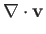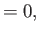(10.40)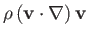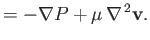(10.41)

As we saw in Sections 10.2 and 10.4, for the case of flow along a straight channel of uniform cross-section,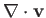and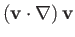are both identically zero, and the governing equations consequently reduce to the simple relation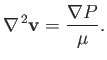(10.42)

Suppose, however, that the cross-section of the channel varies along its length. As we shall demonstrate, provided this variation is sufficiently slow, the flow is still approximately described by the previous relation.

Consider steady, two-dimensional, viscous flow, that is predominately in the-direction, between two plates that are predominately parallel to the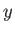-plane. Let the spacing between the plates,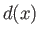, vary on some length scale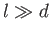. Suppose that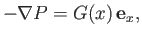(10.43)

where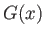also varies on the same lengthscale. Assuming that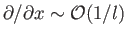and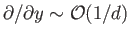, it follows from Equation (10.40) that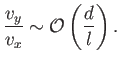(10.44)

Hence,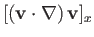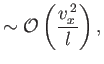(10.45)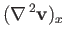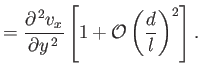(10.46)

The-component of Equation (10.41) reduces to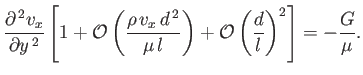(10.47)

Thus, if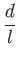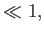(10.48)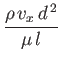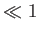(10.49)

--in other words, if the channel is sufficiently narrow, and its cross-section varies sufficiently slowly along its length--then Equation (10.47) can be approximated as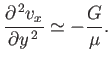(10.50)

This, of course, is the same as the equation governing steady, two-dimensional, viscous flow between exactly parallel plates. (See Section 10.2.) Assuming that the plates are located at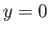and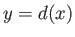, and making use of the analysis of Section 10.2, the appropriate solution to the previous equation is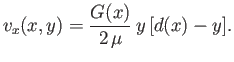(10.51)

The volume flux (per unit width) of fluid between the plates is thus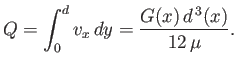(10.52)

However, for steady incompressible flow, this flux must be independent of, which implies that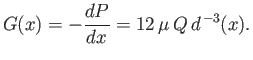(10.53)

Suppose that a constant difference in effective pressure,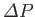, is established between the fixed points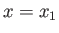and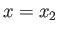, where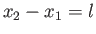. Integration of the previous equation between these two points yields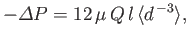(10.54)

where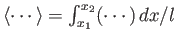. Hence, the volume flux (per unit width) of fluid between the plates that is driven by the effective pressure difference becomes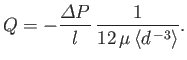(10.55)

Moreover, the effective pressure gradient at a given point is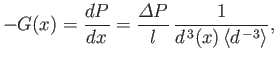(10.56)

which allows us to determine the velocity profile at that point from Equation (10.51). Thus, given the average effective pressure gradient,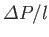, and the variable separation,, we can fully specify the flow between the plates.

Using analogous arguments to those employed previously, but adapting the analysis of Section 10.4, rather than that of Section 10.1, we can easily show that steady viscous flow down a straight pipe of circular cross-section, whose radiusvaries slowly with distance,, along the pipe, is characterized by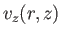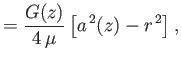(10.57)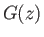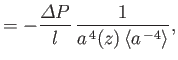(10.58)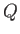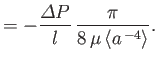(10.59)

Here,is the volume flux of fluid down the pipe,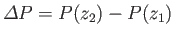,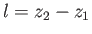, and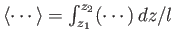. The approximations used to derive the previous results are valid provided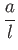(10.60)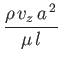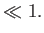(10.61)Next: Lubrication Theory Up: Incompressible Viscous Flow Previous: Taylor-Couette Flow
Richard Fitzpatrick 2016-01-22